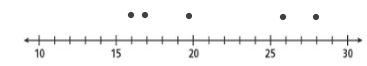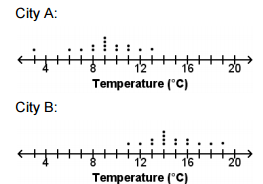# Data AnalysisIn this lesson, you will extend your knowledge of statistical data by learning to read and interpret box and whisker plots and other data representations. Box plots are one form of graphing that can be used to analyze how data is distributed. Dot Plots are not as specific, but they can also be used to compare two sets of data.

In this lesson, you will learn how to compare two data sets and continue to expand your understanding of graphs, mean and median, and interquartile range.

First, let us review the creation of a box-and-whisker plot. A box-and-whisker plot, also known as a box plot or five-number summary, uses a number line to show the distribution of a set of data. The data is divided into four parts known as quartiles.

The highest and lowest quarter of the data is displayed as whiskers and the middle two quarters of the data is displayed as a box. The interquartile range of a data set is the difference between the lower and upper quartiles and demonstrates the side of the data spread around the median. (You can review the lesson on Day 42 if you need a reminder about quartiles and interquartile range.)

### Example: Box Plots

Use the data to create a box-and-whisker plot.

25, 16, 20, 22, 18, 27, 16, 19, 28

Step 1: Order the data from least to greatest. Then, find the lower and upper extremes, the median, and the lower and upper quartiles.Step 2: Draw a number line and plot a point for each value from step one.Step 3: Draw a box from the lower to the upper quartile. Inside the box, draw vertical lines through the median, and draw horizontal lines, “whiskers” to each of the extremes.Now, let’s add another layer to our box and whisker by plotting two sets of data on one number line. The same steps are followed each time a box-and-whisker plot is created. We will begin with a word problem that requires comparison of data.Dee is researching bird-watching tours for her upcoming trip to the coast. She has found two companies that offer the same type of tour for about the same price and is having a difficult time trying to decide which tour is best. She created a box and whisker plot for each company to help her with the comparison.

Examine the two box plots on the number line. The median number of birds seen on Burt’s Bird-Watching tour is 54 and the median birds seen on Friendly Feathers are 51. Remember that the length of the box indicates a greater interquartile range so we can see that Burt’s has the greater range.

Dee knows that the interquartile range on the Friendly Feathers tour is smaller, which means that the data is more predictable, and she wants to make sure that she sees lots of different birds so she decides to go on the tour with Friendly Feathers.

For more in depth information on creating and reading a box-and-whisker, take a look at this video.

Finally, here is a lesson on when you might want to use a Box and Whisker Plot to accurately represent your data.

You can also compare data using a dot plot. You have learned in prior years to read and analyze a single dot plot. In this example, you will see how data can be easily compared between the two plots.

### Example: Dot Plots

A middle school girls’ track coach records the time, in minutes, for each player on the seventh grade team and the eighth grade team to run one mile. The dot plots below show the results. Which of the following statements best compares the median times for the two teams?You can determine that the median time for the eighth grade players is less than the median time for the seventh grade players, but the difference is small compared to the ranges of the data sets.

Now, let us examine overlapping plots. The dot plots below show the high temperatures, in degrees Celsius, of two cities for the past 15 days. Do more than half of the data points from the two cities overlap?No. Only 4 of the 15 data points from each data set overlap the other set. At least 8 data points from one set would have to overlap the other set for more than half of the data points to overlap. This tells us that the means probably are not very close. Now, let us take a look at plots that have a large area of overlap.

Deanna is comparing the number of people per household in two neighborhoods. She takes a random sample of 20 households from each neighborhood and displays her results on the dot plots shown. Does this provide evidence that the mean number of people per household on School Street and on Main Street are different?Actually, since there is a large area of overlap between the sample dot plot for School Street and the sample dot plot for Main Street, it is likely that the means are about the same.

Remember that measures of central tendency can be used to interpret the average of a data set. Measures of central tendency include mean, median, and mode. On the other hand, the spread of a data set is a measure of variation. Quartiles, interquartile ranges, and ranges help measure the spread of the data.

## Box and Whisker Plot Data Analysis

The average monthly rainfall (in inches) in Springville and in Centerville was recorded each month for one year. The box-and-whisker plots display the data.1. Use the box-and-whisker plots to find the median monthly rainfall in Springville to the nearest tenth of an inch.
2. Use the Springville median to predict how much rain Springville receives in 1 year.
3. Which City has the more predictable amount of rainfall?
4. Which City recorded the largest amount of rainfall?
5. About nine months out of the year, Springville receives more than how many inches of rainfall?
6. About nine months out of the year, Centerville receives less than how many inches of rain?
7. Which city recorded the least amount of rainfall in a single month?(source)